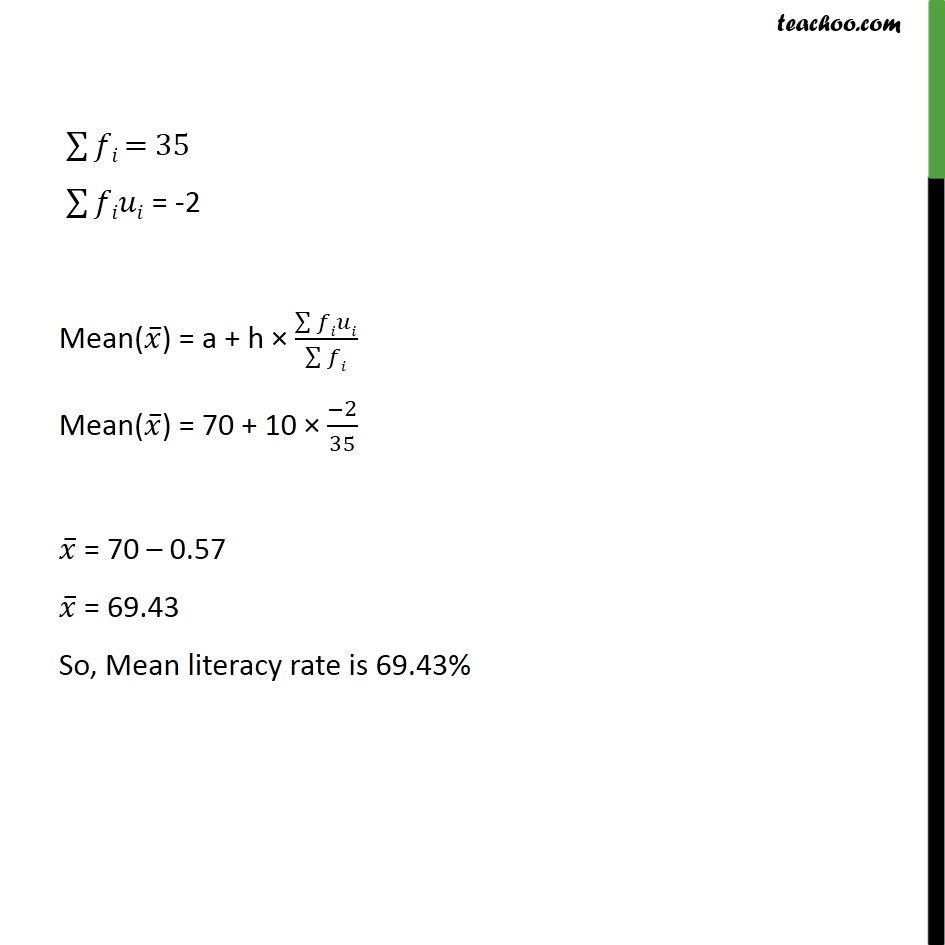1. Chapter 14 Class 10 Statistics
2. Concept wise
3. Mean

Transcript

Ex 14.1, 9 The following table gives the literacy rate (in percentage) of 35 cities. Find the mean literacy rate. Literacy rate (in %) Number of cities 45 – 55 3 55 – 65 10 65 – 75 11 75 – 85 8 85 – 95 3 Class mark (xi) (45 + 55)/2=50 (55 + 65)/2=60 (65 + 75)/2=70 (75 + 85)/2=80 (85 + 75)/2=90 50 - 70 =-20 60 - 70 = -10 70 - 70 =0 80 - 70 =10 90 - 70 =20 Mean(𝑥 ̅) = a + h × (∑▒𝑓𝑖𝑢𝑖)/(∑▒𝑓𝑖) Where a = assumed mean= 70 h = Class interval = 55 – 45 = 10 & ui = (𝑥𝑖 −𝑎)/ℎ ∑▒𝑓𝑖 = 35 ∑▒𝑓𝑖𝑢𝑖 = -2 Mean(𝑥 ̅) = a + h × (∑▒𝑓𝑖𝑢𝑖)/(∑▒𝑓𝑖) Mean(𝑥 ̅) = 70 + 10 × (−2)/35 𝑥 ̅ = 70 – 0.57 𝑥 ̅ = 69.43 So, Mean literacy rate is 69.43%

Mean